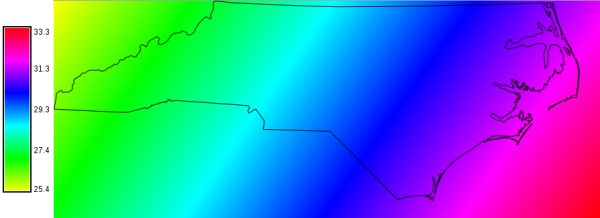## NAME

r.sunhours - Calculates solar elevation, solar azimuth, and sun hours.
Solar elevation: the angle between the direction of the geometric center of the sun's apparent disk and the (idealized) horizon. Solar azimuth: the angle from due north in clockwise direction.

## KEYWORDS

raster, solar, sun energy, sun position

## SYNOPSIS

r.sunhours
r.sunhours --help
r.sunhours [-ts] [elevation=name] [azimuth=name] [sunhour=name] year=integer [month=integer] day=integer [hour=integer] [minute=integer] [second=integer] [--overwrite] [--help] [--verbose] [--quiet] [--ui]

### Flags:

-t
Time is local sidereal time, not Greenwich standard time
-s
Do not use SOLPOS algorithm of NREL
--overwrite
Allow output files to overwrite existing files
--help
Print usage summary
--verbose
Verbose module output
--quiet
Quiet module output
--ui
Force launching GUI dialog

### Parameters:

elevation=name
Output raster map with solar elevation angle
Name for output raster map
azimuth=name
Output raster map with solar azimuth angle
Name for output raster map
sunhour=name
Output raster map with sunshine hours
Sunshine hours require SOLPOS use and Greenwich standard time
year=integer [required]
Year
Options: 1950-2050
month=integer
Month
If not given, day is interpreted as day of the year
Options: 1-12
day=integer [required]
Day
Options: 1-366
hour=integer
Hour
Options: 0-24
Default: 12
minute=integer
Minutes
Options: 0-60
Default: 0
second=integer
Seconds
Options: 0-60
Default: 0

## DESCRIPTION

r.sunhours calculates sun elevation and sun azimuth angles for the given time of day and each grid cell in the current region. Additionally, the photoperiod (sunshine hours on flat terrain) can be calculated.

Sun elevation, height, height angle, or solar altitude angle is the angle in degrees between the horizon and a line that points from the site towards the centre of the sun.

The sun azimuth angle is here defined as the azimuth angle in degrees of the sun from due north in a clockwise direction.

The time used here is defined such that 12:00 (high noon) is the time when the sun has reached its highest point in the sky at the current site, unless the -t flag is used in which case time is interpreted as Greenwich standard time.

If a sunhour output map is specified, the module calculates sunshine hours for the given day. This option requires both Greenwhich standard time and the use of the SOLPOS algorithm by NREL.

## NOTES

To consider also cast shadow effects of the terrain, r.sun has to be used.

## EXAMPLES

### Calculating a sun elevation angle map

Calculate the sun elevation angle map for 2010-10-11 at 14:00h solar time:
```# set computational region to North Carolina state extent
g.region n=318500 s=-16000 w=124000 e=963000 res=500 -p
r.sunhours elevation=sun_elev year=2010 month=10 day=11 hour=14 minute=00

# visualize
d.mon wx0
d.rast sun_elev
d.vect nc_state type=boundary
d.legend sun_elev -s
```Sun angle map (in degree) of North Carolina for the 2010-10-11 at 14:00h solar time

### Calculate map of photoperiod (insolation time)

Calculate photoperiod of day-of-year 001 (1st January) of 2012 for the current computational region, ignoring cast shadow effects of the terrain:
```g.region -p
r.sunhours sunhour=photoperiod_doy_001 year=2012 day=1
```

## Acknowledgements

Acknowledgements: National Renewable Energy Laboratory for their SOLPOS 2.0 sun position algorithm.